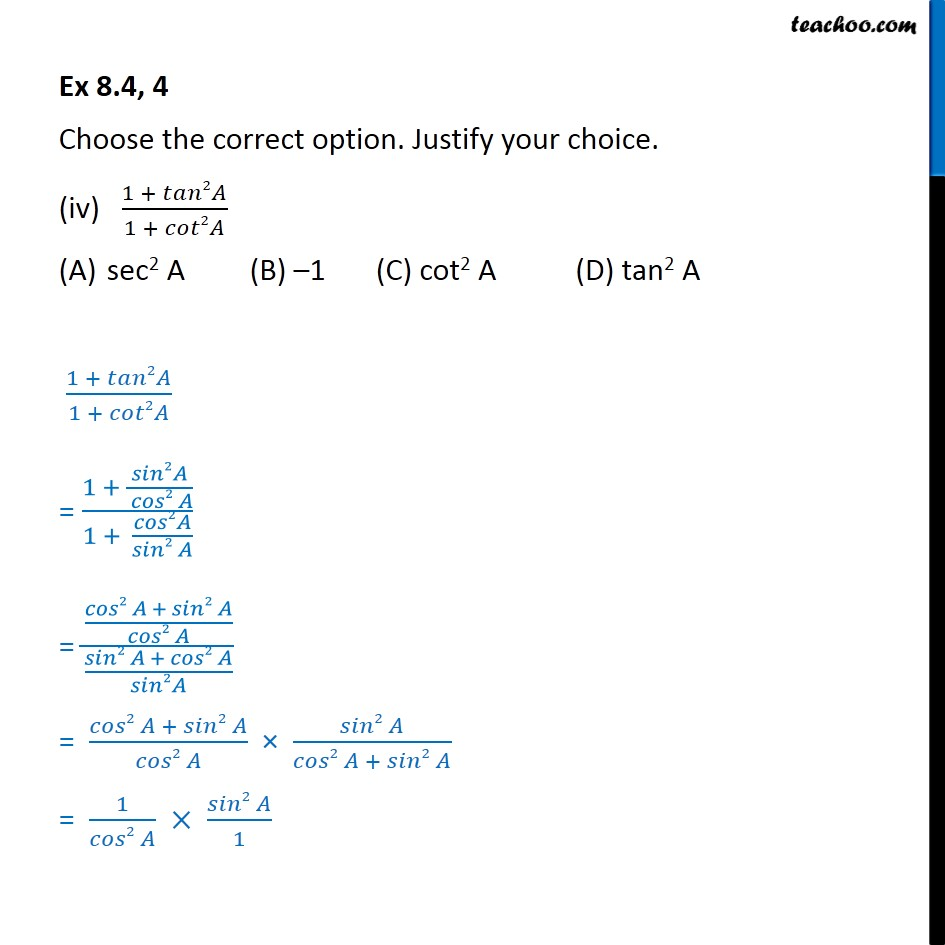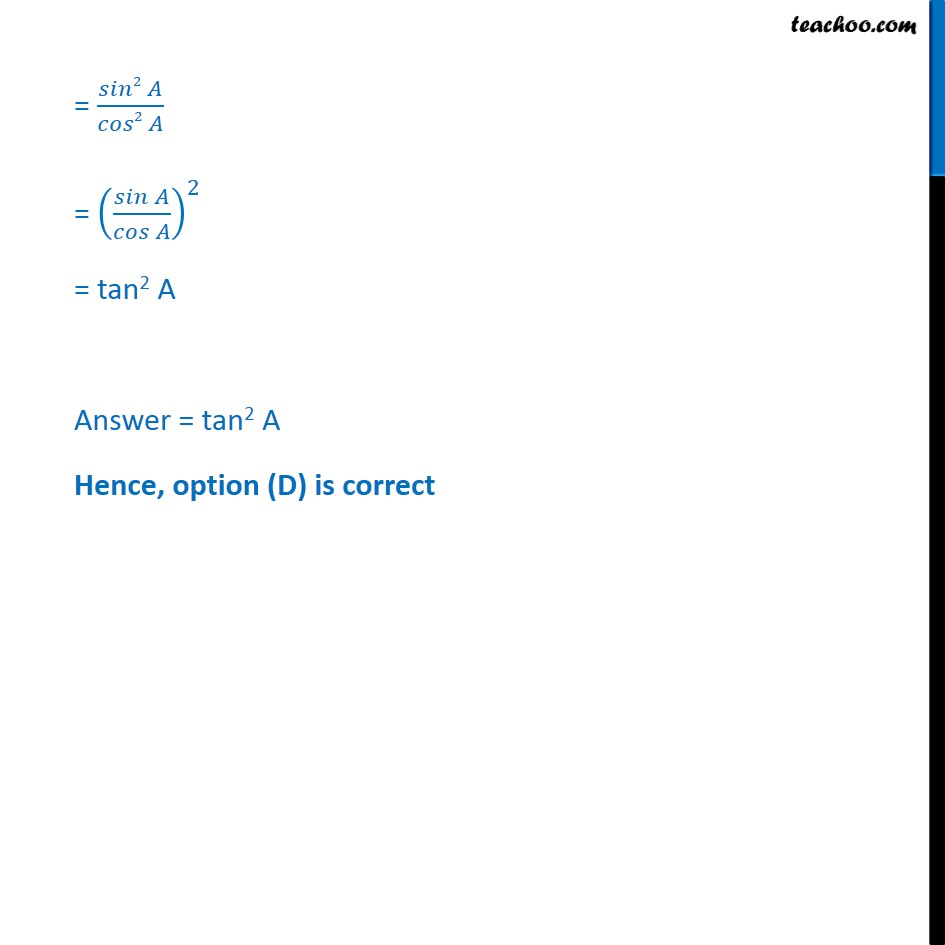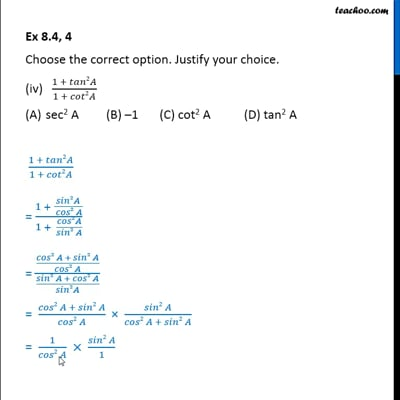Ex 8.4

Chapter 8 Class 10 Introduction to Trignometry
Serial order wiseThis video is only available for Teachoo black users

Get live Maths 1-on-1 Classs - Class 6 to 12

### Transcript

Ex 8.4, 4 Choose the correct option. Justify your choice. (iv) (1 + 𝑡𝑎𝑛2𝐴)/(1 + 𝑐𝑜𝑡2𝐴) sec2 A (B) –1 (C) cot2 A (D) tan2 A (1 + 𝑡𝑎𝑛2𝐴)/(1 + 𝑐𝑜𝑡2𝐴) = (1 + 𝑠𝑖𝑛2𝐴/( 𝑐𝑜𝑠2 𝐴))/(1 + 𝑐𝑜𝑠2𝐴/(𝑠𝑖𝑛2 𝐴)) = ((𝑐𝑜𝑠2 𝐴 + 𝑠𝑖𝑛2 𝐴)/(𝑐𝑜𝑠2 𝐴))/((𝑠𝑖𝑛2 𝐴 + 𝑐𝑜𝑠2 𝐴)/𝑠𝑖𝑛2𝐴) = (𝑐𝑜𝑠2 𝐴 + 𝑠𝑖𝑛2 𝐴)/(𝑐𝑜𝑠2 𝐴) × (𝑠𝑖𝑛2 𝐴)/(𝑐𝑜𝑠2 𝐴 + 𝑠𝑖𝑛2 𝐴) = 1/(𝑐𝑜𝑠2 𝐴) × (𝑠𝑖𝑛2 𝐴)/1 = (𝑠𝑖𝑛2 𝐴)/(𝑐𝑜𝑠2 𝐴) = ((𝑠𝑖𝑛 𝐴)/(𝑐𝑜𝑠 𝐴))^2 = tan2 A Answer = tan2 A Hence, option (D) is correct